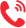• 专业名称
• 所属专业
• 学制
• 学费(元)
• 地区
• 简章详情
• 咨询
• 报名

• 专业名称
• 所属专业
• 学制
• 学费(元)
• 地区
• 简章详情
• 咨询
• 报名

• 专业名称
• 所属专业
• 学制
• 学费(元)
• 地区
• 简章详情
• 咨询
• 报名

• 专业名称
• 所属专业
• 学制
• 学费(元)
• 地区
• 简章详情
• 咨询
• 报名

• 专业名称
• 所属专业
• 学制
• 学费(元)
• 地区
• 简章详情
• 咨询
• 报名

• 专业名称
• 所属专业
• 学制
• 学费(元)
• 地区
• 简章详情
• 咨询
• 报名

• 专业名称
• 所属专业
• 学制
• 学费(元)
• 地区
• 简章详情
• 咨询
• 报名

• 专业名称
• 所属专业
• 学制
• 学费(元)
• 地区
• 简章详情
• 咨询
• 报名

• 专业名称
• 所属专业
• 学制
• 学费(元)
• 地区
• 简章详情
• 咨询
• 报名

• 专业名称
• 所属专业
• 学制
• 学费(元)
• 地区
• 简章详情
• 咨询
• 报名

• 专业名称
• 所属专业
• 学制
• 学费(元)
• 地区
• 简章详情
• 咨询
• 报名

• 专业名称
• 所属专业
• 学制
• 学费(元)
• 地区
• 简章详情
• 咨询
• 报名

• 专业名称
• 所属专业
• 学制
• 学费(元)
• 地区
• 简章详情
• 咨询
• 报名

• 专业名称
• 所属专业
• 学制
• 学费(元)
• 地区
• 简章详情
• 咨询
• 报名

• 专业名称
• 所属专业
• 学制
• 学费(元)
• 地区
• 简章详情
• 咨询
• 报名

## 联系方式400-101-7010

400-101-7010